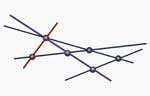Skip to content →After yesterday’s post I had to explain today what
point-modules and line-modules are and that one can really
describe them as points in a (commutative) variety. Seemingly, the
present focus on categorical methods scares possibly interested students
away and none of them seems to know that this non-commutative projective
algebraic geometry once dealt with very concrete examples.
Let
us fix the setting : A will be a quadratic algebra, that is, A is
a positively graded algebra, part of degree zero the basefield k,
generated by its homogeneous part A_1 of degree one (which we take to be
of k-dimension n 1) and with all defining relations quadratic in these
generators. Take m k-independent linear terms (that is, elements of A_1)
: l1,…,lm and consider the graded left A-module

L
= A/(Al1 + ... + Alm)

Clearly, the Hilbert series of this
module (that is, the formal power series in t with coefficient of t^a
the k-dimension of the homogeneous part of L of degree a) starts off
with

Hilb(L,t) = 1  + (n+1-m) t  + ...

and
we call L a linear d-dimensional module if the Hilbert series is
the power series expansion of

1/(1-t)^{d +1} = 1  + (d+1)t   +(d
+1)(d +2)/2 t^2   ... 

In particular, if d=0 (that is, m=n) then L
is said to be a point-module and if d=1 (that is, m=n-1) then L
is said to be a line-module. To a d-dimensional linear module L
one can associate a d-dimensional linear subspace of ordinary (that is,
commutative) projective n-space P^n. To do this, identify

P^n
= P(A 1^*)

the projective space of the n 1 dimensional space of
linear functions on the homogeneous part of degree one. Then each of the
linear elements li determines a hyperplane V(li) in P^n and the
intersection of the m hyperplanes V(l1),…,V(lm) is the wanted
subspace. In particular, to a point-module corresponds a point in
P^n and to a line-module a line in P^n. So, where
is the non-commutativity of A hidden? Well, if P is a point-module

P
= P0  + P1 +  P2   +... 

(with all components P_a one dimensional)
then the twisted module

P' = P1 +  P2  + P3  + ...

is
again a point-module and the map P–>P’ defines an automorphism on the
point variety. In low dimensions, it is often possible to
reconstruct A from the point-variety and automorphism. In higher
dimensions, one has to consider also the higher dimensional linear
modules.
When I explained all this (far clumsier as it was a
long time since I worked with this) I was asked for an elementary text
on all this. ‘Why hasn’t anybody written a book on all this?’ Well,
Paul Smith wrote such a book so have a look at his
homepage. But then, it turned out that the version one can download from
one of his course pages is a more recent and a lot more
categorical version. There is no more sight of a useful book on
non-commutative projective spaces and their linear modules which might
give starting students an interesting way to learn some non-commutative
algebra and the beginnings of algebraic geometry (commutative and
non-commutative). So, hopefully Paul still has the old version around
and will make it available… The only webpage on this I could find in
short time are the slides of a talk by Michaela Vancliff.

### Similar Posts:

Published in featured

## Comments

This site uses Akismet to reduce spam. Learn how your comment data is processed.